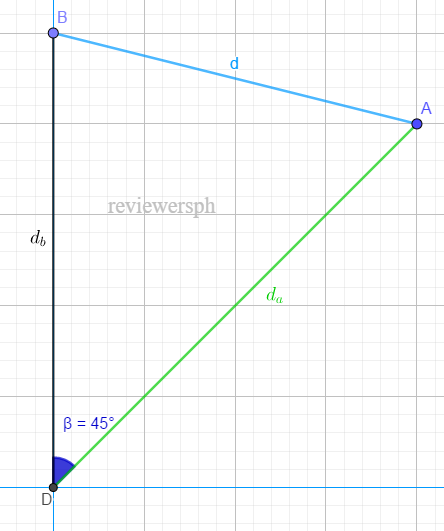### Math Notes

Subjects

#### Trigonometry Solutions

##### Topics || Problems

Two ships leave a dock at the same time. One sail northeast at the rate of 8.5 miles an hour, the other sails north at the rate of 10 miles an hour. How far apart are they at the end of 2 hours.

Let $$A$$ be the first ship and $$B$$ be the second ship.

Velocity, $$V = \frac{distance}{time}$$

After $$2$$ hours ship $$A$$ travelled $$d_a = 8.5(2) = 17 ~ mi$$ while ship $$B$$ travelled $$d_b = 10 (2) = 20 ~mi$$The distance between the ships after 2 hours, can be calculated by $$cos$$ law

$$d^2 = d_{b}^2 + d_{a}^2 - 2d_ad_b \cos 45$$

$$d^2 = 20^2 + 17^2 - 2(20)(17) \cos 45$$

$$d^2 = 208.17$$

$$d = 14.43 ~mi$$